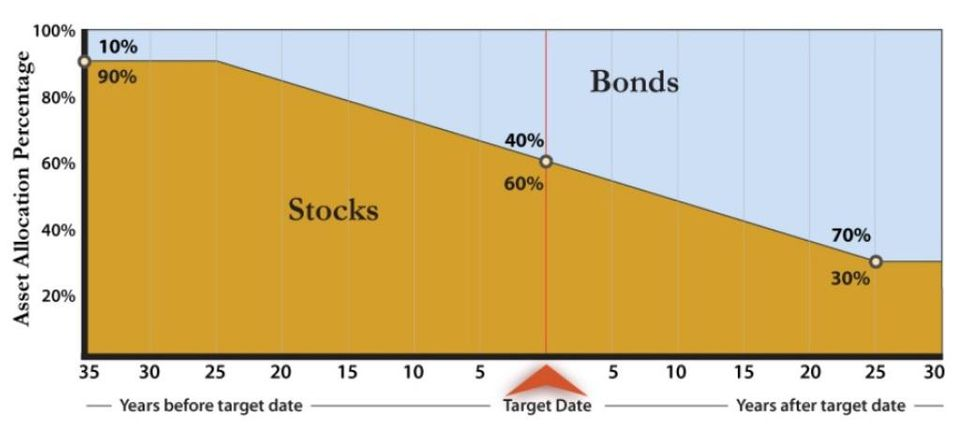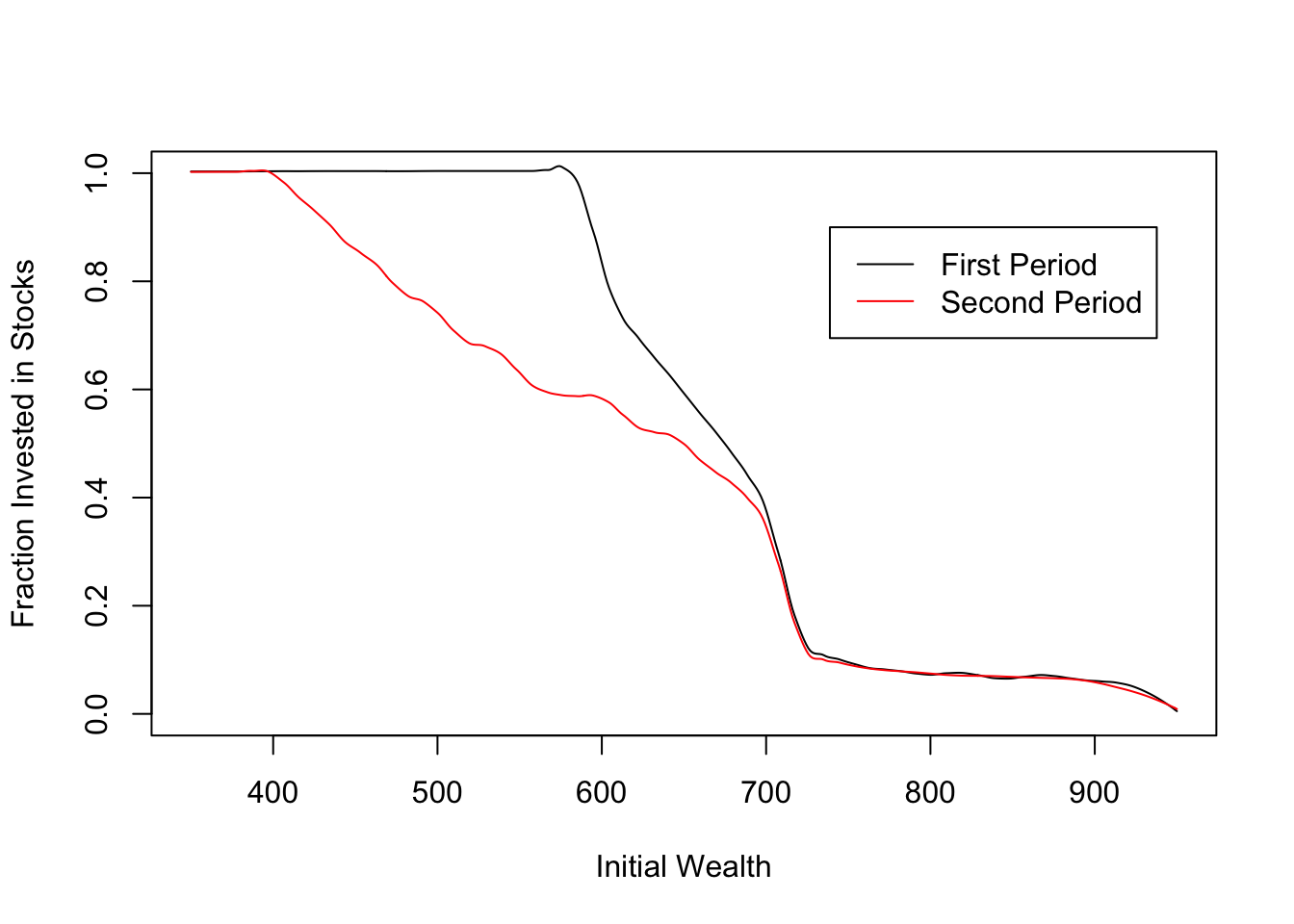# Do Target Date Funds Make Sense?

## Motivation

A common piece of investing advice goes as follows:1

Stock-based funds have higher long-term return potential but also have relatively high volatility. On the other hand, bond funds are more stable, especially in terms of income, but don’t have the long-term growth potential of stocks. Therefore, it’s generally suggested that younger investors put most of their money into stocks and gradually shift their investments into bonds as they get closer to retirement.

For investors that don’t want to actively manage their retirement portfolio, companies such as Vanguard have introduced “Target Date Funds” (TDFs), which are marketed as a “one stop shop” for retirement investing. Vanguard’s explanation states, “managers gradually shift each fund’s asset allocation to fewer stocks and more bonds so the fund becomes more conservative the closer you get to retirement” (see Figure 1). My employer plan goes straight to a TDF, and I am not alone: according to this Forbes article, most employer-sponsored retirement funds default to TDFs, and it is predicted that 88% of retirement plan contributions will flow into TDFs by 2019.

TDFs are popular, but does their approach actually make sense? In this post, I investigate that question, taking as given the assertion that stocks are riskier than bonds, but have a higher expected return. I start by introducing a simple model that will frame my thinking. In the end, I conclude that (1) starting with much of your money in stocks likely makes sense for many investors, but (2) the “set it and forget it” strategy of letting your TDF automatically shift this money to bonds likely does not.Figure 1: Mix of stocks and bonds in a Target Date Fund over time. Source: US Department of Labor investment bulletin on TDFs.

An investor starts with $1. In each period $$t$$, she may place a fraction $$\alpha_t$$ of her wealth in a risky asset, with (unknown) return $$r_t$$; the remaining $$1 - \alpha_t$$ fraction of her wealth goes into a safe asset with known return $$s$$. Given returns $$r_t$$ and investment decisions $$\alpha_t$$, her per-period wealth $$W_t$$ evolves as follows: $W_{t+1} = (\alpha_t r_t + (1 - \alpha_t) s) W_t.$ Assume that $$s \geq 1$$ and $$r_t$$ are iid draws from a known distribution $$G$$, with $$E[r_t] > s$$ and $$P(r_t < s) > 0$$: that is, the risky asset has higher average return, but is sometimes worse than the safe one. Our investor plans to retire in period $$T$$. Her final utility is given by $$U(W_T)$$ for some known and weakly increasing function $$U$$, and she invests to maximize her expected utility. ## Being Contrarian I start by presenting evidence against the conventional wisdom that investors should gradually shift from stocks to bonds. Proposition. If investors maximize expected wealth $$(U(x) = x)$$, then it is optimal to select $$\alpha_t = 1$$ for all $$t$$. Of course, most investors don’t aim to maximize expected wealth. Generally, money is assumed to have diminishing marginal returns. One standard assumption that captures this effect is to take $$U(x) = \log(x)$$, implying that in order to increase the utility of two different investors by equal amounts, it is necessary to increase their wealth by equal percentages. In this case, it may make sense to invest in a mix of bonds and stocks, but the optimal mix does not depend on one’s wealth level or the time until retirement. Proposition. If investors maximize the expected logarithm of wealth $$(U(x) = \log(x))$$, then there exists $$\alpha \in [0,1]$$ such that it is optimal to select $$\alpha_t = \alpha$$ for all $$t$$. Of course, $$U(x) = \log(x)$$ is still one particular functional form. However, it turns out that for any utility function $$U$$, committing to any particular trajectory of the stock-bond mix is no better than committing to the reverse. Proposition. If investors follow a non-adaptive policy2 $$\alpha = (\alpha_1, \ldots, \alpha_{T-1})$$, then the distribution of $$W_T$$ is invariant to a permutation in $$\alpha$$. To put this in plain English, let’s compare two simple strategies: A. 25 years with 80% stocks, followed by 10 years with 80% bonds. B. 10 years with 80% bonds, followed by 25 years with 80% stocks. While traditionalists will tell you that strategy A is less risky than B, this is not true! The probability of having less than $$D$$ dollars in savings upon retirement is identical under these strategies, for any $$D$$. ## Adaptive Investment The preceding section establishes that among non-adaptive (“set it and forget it”) investment policies, there is no reason that the investment in stocks should decrease over time. Why, then, is this advice so prevalent? One important observation is that I can adjust my behavior based on how my portfolio is doing. For example, I might initially invest in stocks, and then check my portfolio’s value several years later. If the portfolio has done well, I will shift my money to bonds, to lock in my savings. If the portfolio has tanked, shifting it to bonds will still leave me too poor to retire, so I keep my money in stocks and hope for good returns.3 This suggests that the optimal strategy will start with risky assets, to resolve uncertainty early and be able to respond accordingly. We can test this intuition numerically using dynamic programming. The following code is for a two-period example.4 In this example, on average, the investor shifts money from stocks to bonds in the second period, but in some cases she actually does the reverse.5 #Given vectors X,Y such that X is increasing and Y = g(X), returns a discretized function approximating g approximate = function(X,Y){ return(Vectorize(function(x){ i = max(c(which(X < x),1)) return(Y[i]) #Could also do an average of Y[i] and Y[i+1], or linear interpolation })) } #Given current wealth w, return from safe asset s, value function V and return from risky asset (r,p), computes expected utility from any given portfolio mix alpha # p is a probability vector of the same length as r, and s and w are scalars. expectedUtility = function(w,s,r,p,V){ return(Vectorize(function(alpha){ return(sum(V((alpha*r+(1-alpha)*s)*w)*p)) })) } s = 1 #return from safe asset r = .6 + c(0:10)/10 #possible returns from risky asset p = rep(1/length(r),length(r)) #p[i] is probability of return r[i] #Utility of wealth function #U for plot 1 U = Vectorize(function(x){return(1/(1+exp(-a*(x-b))))}) #Other possible utility functions #U = Vectorize(function(x){return(sqrt(x/b)/2)}) #Target of 650 #U = Vectorize(function(x){if(x>=650) return(1); return(0)}) a = 0.02; b= 650; N = 250 W = b+6*rep(-N:N)/(a*N) #Different levels of wealth, in a range relevant to U #Solving a two-period problem T = 2 V = matrix(0,nrow = T+1,ncol=length(W)) #V[t+1,i] gives value of having W[i] dollars with t periods remaining Alpha = matrix(0,nrow = T+1,ncol=length(W)) #Alpha[t+1,i] gives optimal portfolio wit W[i] dollars and t periods remaining V[1,] = U(W) for(t in 1:T){ for(i in 1:length(W)){ EU = expectedUtility(W[i],s,r,p,approximate(W,V[t,]))(c(0:N)/N) #Taking this approach because the optimize function works poorly for locally flat functions V[t+1,i] = max(EU) Alpha[t+1,i] = which(EU==max(EU))/N #soln = optimize(expectedUtility(W[i],s,r,p,approximate(W,V[t,])),interval=c(0,1),maximum=TRUE) #Alpha[t+1,i] = soln$maximum  #What is optimal alpha, given t remaining periods and wealth level W[i]?
#V[t+1,i] = soln\$objective #What is expected value, given t remaining periods and wealth level W[i]?
}
}
#Two period model -- compare alpha in first period to average alpha in second period
AlphaSecondPdAvg = rep(0,length(W))  #AlphaSecondPdAvg[i] gives average alpha in second period, when investor has W[i] with two periods to go and behaves optimally
alphafn = approximate(W,Alpha[2,])  #alphafn(w) says what alpha to choose given wealth of w and one period remaining
for(i in 1:length(W)){
AlphaSecondPdAvg[i] = sum(alphafn((Alpha[3,i]*r+(1-Alpha[3,i])*s)*W[i])*p)  #Alpha[3,i] is optimal alpha, given wealth W[i] with two periods remaining
}
plot(NULL,xlab='Initial Wealth',xlim=c(W,W[length(W)]),ylab='Fraction Invested in Stocks',ylim=c(0,1))
lines(W,predict(loess(Alpha[3,]~W,span=0.1)),col='black')
lines(W,predict(loess(AlphaSecondPdAvg~W,span=0.1)),col='red')
legend(x=W[N*1.3],y=0.9,legend = c('First Period','Second Period'),col=c('black','red'),lty=c(1,1))## Discussion

So, does it make sense to gradually shift assets from stocks to bonds as retirement approaches?

My answer is a qualified yes. An investor who actively manages her portfolio to prepare for retirement might reasonably expect to see the percentage of stocks decline over the years. The reason for this is that investing in stocks early provides information which can be used to make future decisions. In the model, the only decision is the mix of stocks and bonds, but in reality an investor may make other adjustments in response to early returns, such as changing consumption behavior or deciding to work for a few extra years.

However, the shift to bonds only happens on average, and only makes sense if the investor is looking at her retirement account and adjusting her behavior accordingly. This suggests that sales pitch for Target Date Funds doesn’t really make sense – they cater to investors who want to set aside money and forget about it until retirement, but adopt a “glide” strategy that can’t easily be justified for these investors.

As one Investopedia article states,

One retiree may have enough money on hand to invest strictly in bonds and other fixed-income securities. Another, requiring both growth and income, may need an equity component to keep the portfolio on track. A fund that meets the needs of one of these investors is unlikely to meet the needs of the other.

Will I redirect my contributions away from the Target Date Fund? Not right away – it’s mostly stocks right now, as it likely should be. But don’t be surprised if I end up shifting the money more actively at some point in the future.

Questions for readers: Is your money in a TDF? Why do you think these are so common? And what is the best counter-argument to the ideas above?

1. Quote taken from the Motley Fool.

2. I say that a policy is non-adaptive if $$\alpha_t$$ is independent of the vector of returns $${\bf r}$$.

3. This relates to my post on 2-point Conversions in the NFL. There, the best strategy is to go for two after the first touchdown. If that succeeds, the team takes the “safe” strategy (an extra point) on its second touchdown. If the first two-point try fails, the team gambles on another two-pointer to try to catch up. Thus, in both settings, it pays to take the riskier decision first, but only if you are going to use the information that you get to inform future decisions!

4. No apologies for the limitations of my code, as writing non-robust code is one of the joys of being an academic.

5. I would be curious establish conditions under which the investor expects to hold a higher percentage of stocks in the first period than the second. One tricky aspect is that while the value function is unique, the optimal policy may not be. There sometimes exist optimal strategies under which the expected fraction of the portfolio invested in stocks increases over time, but it is conceivable to me that there always exists an optimal strategy under which the investor expects to gradually shift to bonds.